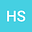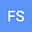loading page

Fractional Nonuniform Multiresolution Analysis in $L^2(\mathbb R)$
•••Hari M. Srivastava
University of Victoria
Author ProfileFirdous A. Shah
University of Kashmir
Author Profile## Abstract

In order to provide a significantly richer representation of non-stationary signals appearing in various disciplines of science and engineering, we introduce here a novel fractional nonuniform multiresolution analysis (FrNUMRA) on the spectrum $\Lambda$ given by $\Lambda = \left\{0,\frac{r}{N}\right\}+2\mathbb{Z}$, where $N \geqq 1$ is an integer and $r$ is an odd integer with $1 \leqq r \leqq 2N-1,$ such that $r$ and $N$ are relatively prime. The necessary and sufficient condition for the existence of nonuniform wavelets of fractional order is derived and an algorithm is also presented for the construction of fractional NUMRA starting from a fractional low-pass filter $h_{0}^{\alpha}$ with appropriate conditions. Moreover, we provide a complete characterization for the biorthogonality of the translates of the scaling functions of two fractional nonuniform multiresolution analyses and the associated fractional biorthogonal wavelet families.

#### Peer review status:ACCEPTED

18 Sep 2020Submitted to Mathematical Methods in the Applied Sciences
19 Sep 2020Submission Checks Completed
19 Sep 2020Assigned to Editor
17 Oct 2020Reviewer(s) Assigned
19 Feb 2021Review(s) Completed, Editorial Evaluation Pending
24 Feb 2021Editorial Decision: Revise Minor
25 Feb 20211st Revision Received
25 Feb 2021Submission Checks Completed
25 Feb 2021Assigned to Editor
25 Feb 2021Editorial Decision: Accept